VBA Course: Using Arrays

Arrays are "variables" that allow many values to be stored. We have already covered this topic in Lesson 3, but now we will go into greater depth ...

Why use arrays?

Imagine that you are trying to write a procedure in which you will need to store 500 values. If you had to create 500 individual variables to do this, it would be extremely difficult. With an array, storing and working with these values will be much easier.

A second reason to use arrays is their "speed". It takes much less time to retrieve data from arrays than from "tables" (made up of cells) on an Excel worksheet ...

So here is an example that will make this all a little clearer ...

On the first worksheet ("DS") you will find: a 5000 row by 3 column data set: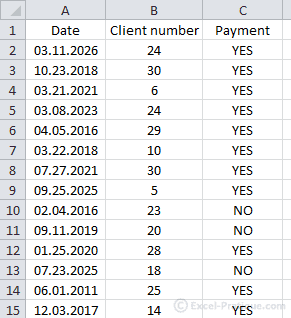On the second worksheet you will find: a summary table which accounts for all the "YES" responses by year and by client: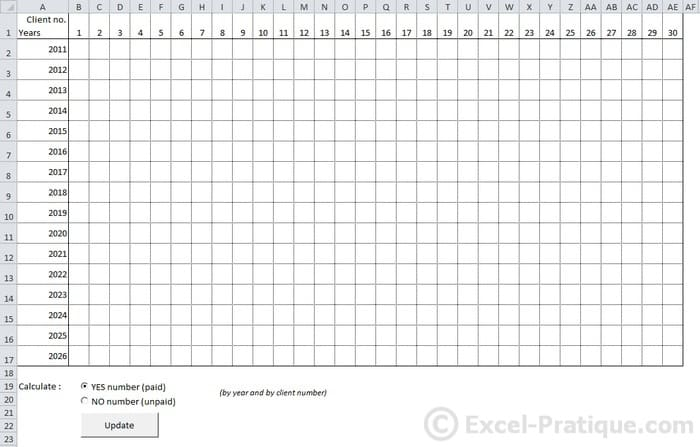In this case, the procedure will use a loop to process the data set and record the number of "YES" responses for each year and each client number, then enter this data into the corresponding cells.

Without using arrays, it would take Excel 131.44 seconds to execute this procedure: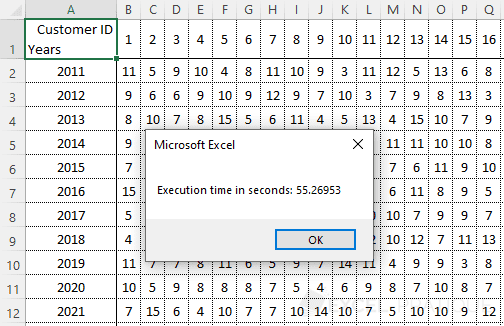But by first storing the data (from worksheet "DS") in an array and then carrying out the same calculations (using the array instead of the data set from worksheet "DS"), it will only take 1.74 seconds for the procedure to execute: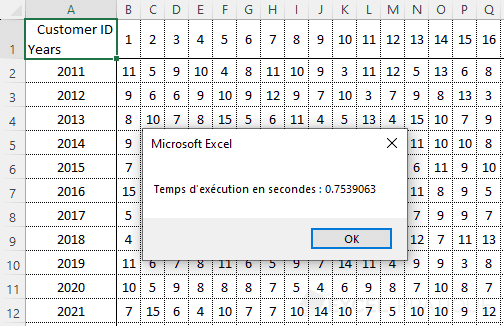And if we decided to optimize the procedure by storing only the data containing "YES" responses in the array (which is about 3/4 of the data), it would take only 1.02 seconds: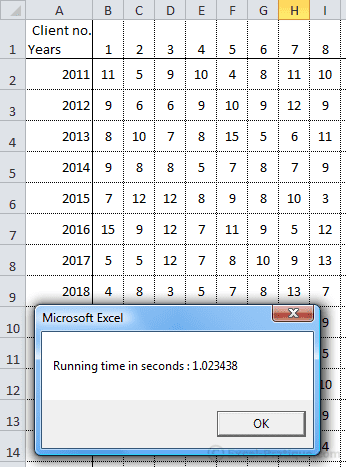This is a good example of how using an array makes it possible to execute a procedure about 128x faster. This improvement would be even greater if we were working with multiple data sets at once.

Now it's easy to see that using arrays makes all the difference in the world.

We'll go into the details of this example at the end of the lesson ...

Declaring an array

Here are a few examples of array declarations (if the first 2 don't make sense to you, reread this):

'Sample declaration of a 1 dimensional array
Dim array1(4)

'Sample declaration of a 2 dimensional array
Dim array2(6, 1)

'Sample declaration of a dynamic array
Dim array3()

If you can't enter fixed values when you declare an array (because they will depend on the size of the data set, for example), leave the parentheses empty.

You don't have to declare a type (string, long, etc.), although in many cases this will slow down the execution of your procedure ...

Storing data in an array

Let's begin by storing some data in an array: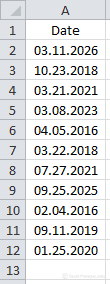We want to store 11 x 1 values in this case, so we need to create a 1 dimensional array:

'Declaration
Dim array_example(10)

Don't forget that array element numbering begins with 0 (this is standard in programming, so it's a good idea to get in the habit of working this way, even though it is actually possible to change this convention in VBA).

Each element in the array will now receive its value:

'Storing values in the array
array_example(0) = Range("A2")
array_example(1) = Range("A3")
array_example(2) = Range("A4")
array_example(3) = Range("A5")
array_example(4) = Range("A6")
array_example(5) = Range("A7")
array_example(6) = Range("A8")
array_example(7) = Range("A9")
array_example(8) = Range("A10")
array_example(9) = Range("A11")
array_example(10) = Range("A12")

You can work with or modify each element of the array as though it were a variable.

Here is an example in which we use array_example(8):

Sub example()
'Declaration
Dim array_example(10)

'Storing values in the array
array_example(0) = Range("A2")
array_example(1) = Range("A3")
array_example(2) = Range("A4")
array_example(3) = Range("A5")
array_example(4) = Range("A6")
array_example(5) = Range("A7")
array_example(6) = Range("A8")
array_example(7) = Range("A9")
array_example(8) = Range("A10")
array_example(9) = Range("A11")
array_example(10) = Range("A12")

'Test 1
MsgBox array_example(8) '=> returns: 02.04.2016

'Changing one of the values
array_example(8) = Year(array_example(8))

'Test 2
MsgBox array_example(8) '=> returns: 2016
End Sub

A For loop would be an effective way to stock the array faster:

'Declaration
Dim array_example(10)

'Storing values in the array
For i = 0 To 10
array_example(i) = Range("A" & i + 2)
Next